# GSEB Solutions Class 12 Maths Chapter 13 Probability Ex 13.2

Gujarat Board GSEB Textbook Solutions Class 12 Maths Chapter 13 Probability Ex 13.2 Textbook Questions and Answers.

## Gujarat Board Textbook Solutions Class 12 Maths Chapter 13 Probability Ex 13.2

Question 1.
If P(A) = $$\frac{3}{5}$$ and P(B) = $$\frac{1}{5}$$, find P(A ∩ B), if A and B are independent events.
Solution:
A and B are independent events. Therefore,
P(A ∩ B) = P(A) × P(B) = $$\frac{3}{5}$$ × $$\frac{1}{5}$$ = $$\frac{3}{25}$$.Question 2.
Two cards are drawn at random and without replacement from a pack of 52 playing cards. Find the probability that both the cards are black.
Solution:
Number of exhaustive cases = 52
Number of black cards = 26
One black card may be drawn in 26 ways
∴ Probability of getting a black card P(A) = $$\frac{26}{52}$$ = $$\frac{1}{2}$$.
After drawing one card, number of cards left = 51.
After drawing a black card, number of black cards left = 25
∴ Probability of getting both the black cards
P(A) P(B/A) = $$\frac{1}{2}$$ × $$\frac{25}{51}$$ = $$\frac{25}{102}$$.

Question 3.
A box of oranges is inspected by examining three randomly selected oranges drawn without replacement.
If all the three oranges are good, the box is approved for sale, otherwise it is rejected.
Find the probability that a box containing 15 oranges, out of which 12 are good and three are bad ones, will be approved for sale.
Solution:
Box contains 12 good and 3 bad oranges. If three oranges selected are good ones, the box is approved for sale.
3 good oranges may be selected in 12C3 ways.
3 oranges out of 15 may be selected in 15C3 ways.
Probability that a box is approved
= Probability of selecting 3 good oranges
= $$\frac{{ }^{12} C_{3}}{{ }^{15} C_{3}}$$ = $$\frac{12×11×10}{15×14×13}$$ = $$\frac{44}{91}$$.Question 4.
A fair coin and an unbiased dice are tossed. Let A be the event ‘head appears on the coin’ and B be the event 3 on dice. Check whether A and B are independent events or not.
Solution:
When a coin is thrown, head or tail will occur.
∴ Probability of getting head P(A) = $$\frac{1}{2}$$.
when a dice is tossed 1, 2, 3, 4, 5, 6 one of them will appear.
∴ Probability of getting 3 = P(B) = $$\frac{1}{6}$$.
When a coin and a dice are tossed, total number of cases are
H1, H2, H3, H4, H5, H6
T1, T2, T3, T4, T5, T6
Head and 3 will occur only in 1 way.
∴ Probability of getting head and 3 = $$\frac{1}{12}$$.
i.e; P(A ∩ B) = $$\frac{1}{12}$$.
∴ P(A) × P(B) = $$\frac{1}{2}$$ × $$\frac{1}{6}$$ = $$\frac{1}{12}$$
∴ P(A ∩ B) = P(A) × P(B)
⇒ Events A and B are independent.

Question 5.
A dice, marked 1, 2, 3 in red and 4, 5, 6 in green is tossed. Let A be the event ‘number is even’ and B be the event ‘number is red’. Are A and B are independent?
Solution:
Even numbers on dice are 2, 4, 6.
∴ Probability of getting an even number = P(A) = $$\frac{3}{6}$$ = $$\frac{1}{2}$$.
There are two colours on the dice viz. red and green.
Probability of getting red colour P(B) = $$\frac{1}{2}$$.
Even number in red colour is 2.
∴ Probability of getting red colour and even number
P(A ∩ B) = $$\frac{1}{6}$$
P(A) P(B) = $$\frac{1}{2}$$ × $$\frac{1}{2}$$ = $$\frac{1}{4}$$ ≠ $$\frac{1}{6}$$ ≠ P(A ∩ B) = $$\frac{1}{6}$$.
⇒ A and B are not independent.Question 6.
Let E and F be the events with P(E) = $$\frac{3}{5}$$, P(F) = $$\frac{3}{10}$$ and P(E ∩ F) = $$\frac{1}{5}$$. Are E and F independent?
Solution:
P(E) = $$\frac{3}{5}$$, P(F) = $$\frac{3}{10}$$
∴ P(E) × P(F) = $$\frac{3}{5}$$ × $$\frac{3}{10}$$ = $$\frac{9}{50}$$
P(E ∩ F) ≠ P(E) × P(F)
∴ The events E and F are not independent.

Question 7.
Given that the events A and B are such that P(A) = $$\frac{1}{2}$$, P(A ∪B) = $$\frac{3}{5}$$ and P(B) = p, If they are
(i) mutually exclusive
(ii) independent.
Solution: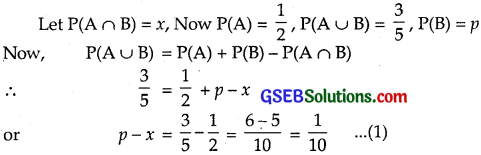(i) When events A and B are mutually exclusive, x = 0
∴ p = $$\frac{1}{10}$$

(ii) When events A and B are independent,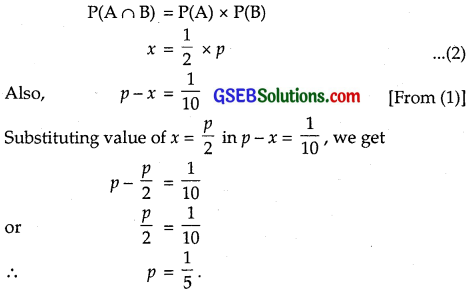Question 8.
Let A and B be independent events P(A) = 0.3 and P(B) = 0.4.
Find :
(i) P(A ∩ B)
(ii) P(A ∪ B)
(iii) P(A/B)
(iv) P(B/A)
Solution:
(i) P(A) = 0.3, P(B) = 0.4
When A and B are independent,
P(A ∩ B) = P(A) × P(B)
= 0.3 × 0.4 = 0.12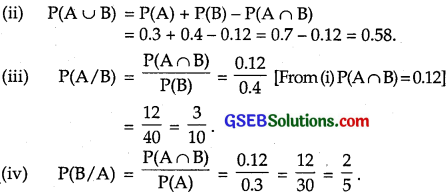Question 9.
If A and B are two events, such that P(A) = $$\frac{1}{4}$$, P(B) = $$\frac{1}{2}$$, P(A ∩ B) = $$\frac{1}{8}$$, find P(not A and not B).
Solution: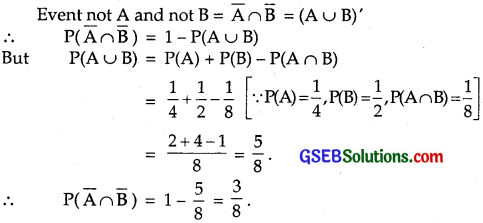Question 10.
Events A and B are such that P(A) = $$\frac{1}{2}$$, P(B) = $$\frac{7}{12}$$ and P(not A or not B) = $$\frac{1}{4}$$.
State whether A and B are independent.
Solution: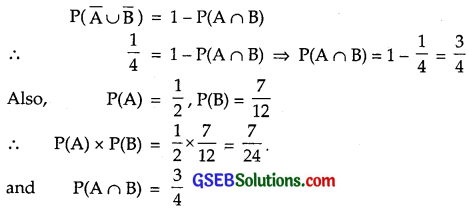⇒ P(A ∩ B) ≠ P(A) × P(B)
⇒ A and B are not independent.Question 11.
Given two independent events A and B such that P(A) = 0.3 and P(B) = 0.6, find
(i) P(A and B)
(ii) P(A and not B)
(iii) P(A or B)
(iv) P(neither A nor B)
Solution:
(i) A and B are independent events.
∴ P(A and B) = P(A ∩ B) = P(A) × P(B)
= 0.3 × 0.6 [∵ P(A) = 0.3, P(B) = 0.6]
= 0.18
∴ P(A and B) = 0.18.

(ii) P(A and not B) = P(A ∩ $$\overline{\mathrm{B}}$$) = P(A) – P(A ∩ B)
= 0.3 – 0.18
[∵ P(A) = 0.3 and P(A ∩ B) = 0.18 from (i)}
= 0.12.

(iii) Here, P(A) = 0.3, P(B) = 0.6 and P(A ∩ B) = 0.18.
∴ P(A ∪ B) = P(A) + P(B) – P(A ∩ B)
= 0.3 + 0.6 – 0.18 = 0.9 – 0.18 = 0.72
⇒ P(A or B) = 0.72.

(iv) P(neither A nor B) = P($$\overline{\mathrm{A}}$$ ∩ $$\overline{\mathrm{B}}$$) = P($$\overline{\mathrm{A}}$$) × P($$\overline{\mathrm{B}}$$)
[When A and B are independent]
∴ P($$\overline{\mathrm{A}}$$ ∩ $$\overline{\mathrm{B}}$$) = P($$\overline{\mathrm{A}}$$) × P($$\overline{\mathrm{B}}$$)
[When A and B are independent]
∴ P($$\overline{\mathrm{A}}$$ ∩ $$\overline{\mathrm{B}}$$) = P($$\overline{\mathrm{A}}$$) × P($$\overline{\mathrm{B}}$$)
= (1 – 0.3) × (1 – 0.6)
= 0.7 × 0.4 = 0.28.Question 12.
A dice is tossed thrice. Find the probability of getting an odd number at least once?
Solution:
If a dice is thrown and an odd number occurs, then it may result in 1, 3, 5.
∴ The probability of getting an odd number in a throw of a dice
= $$\frac{3}{6}$$ = $$\frac{1}{2}$$.
∴ Probability of getting an even number = $$\frac{1}{2}$$.
Now the probability of getting no odd number in a throw of dice 3 times
= Probability of getting an even number in a throw of a dice 3 times
= $$\frac{1}{2}$$ × $$\frac{1}{2}$$ × $$\frac{1}{2}$$ = $$\frac{1}{8}$$.
∴ Probability of getting at least one odd number in a throw of a dice 3 times
= 1 – $$\frac{1}{8}$$ = $$\frac{7}{8}$$.

Question 13.
Two balls are drawn at random with replacement from a box containing 10 black and 8 red balls. Find the probability that
(i) both balls are red.
(ii) first ball is black and second is red.
(iii) one of them is black and other is red.
Solution:
There are 10 black (B) and 8 red (R) balls.
(i) Probability of getting first ball red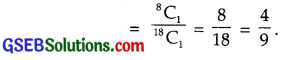Probability of getting second ball also red = $$\frac{4}{9}$$
(since the first ball has been replaced)
⇒ The probability of getting both balls red
= $$\frac{4}{9}$$ × $$\frac{4}{9}$$ = $$\frac{16}{81}$$.

(ii) The probability of getting first ball black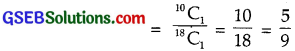The probability of getting second ball red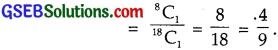∴ Probability of getting first ball black and second ball red (with replacement)
= $$\frac{5}{9}$$ × $$\frac{4}{9}$$ = $$\frac{20}{81}$$.

(iii) One of them is black and other is red may be obtained as BR, RB
∴ Probability of getting first ball black and second red
= $$\frac{10}{18}$$ × $$\frac{8}{18}$$ = $$\frac{5}{9}$$ × $$\frac{4}{9}$$ = $$\frac{20}{81}$$
Probability of getting first ball red and second ball black
= $$\frac{8}{18}$$ × $$\frac{10}{18}$$ = $$\frac{4}{9}$$ × $$\frac{5}{9}$$ = $$\frac{20}{81}$$
∴ Probability of getting one of them black and other red
= $$\frac{20}{81}$$ + $$\frac{20}{81}$$ = $$\frac{40}{81}$$.Question 14.
Problem of solving specific problem independently by A and B are $$\frac{1}{2}$$ and $$\frac{1}{3}$$ respectively.
If both try to solve the problem independently, find the probability that:
(i) The problem is solved.
(ii) Exactly one of them solves the problem.
Solution:
Probability that A solves the problem = $$\frac{1}{2}$$
⇒ Probability that A does not solve the problem P($$\overline{\mathrm{A}}$$)
= 1 – $$\frac{1}{2}$$ = $$\frac{1}{2}$$.
Probability that B solves the problem = $$\frac{1}{3}$$
⇒ Probability that B does not solve the problem
= 1 – $$\frac{1}{3}$$ = $$\frac{2}{3}$$.

(i) Probability that problem is not solved P($$\overline{\mathrm{A}}$$ $$\overline{\mathrm{B}}$$)
= P($$\overline{\mathrm{A}}$$) P($$\overline{\mathrm{B}}$$) = $$\frac{1}{2}$$ × $$\frac{2}{3}$$ = $$\frac{1}{3}$$.
⇒ Probability that problem is solved
= 1 – P($$\overline{\mathrm{A}}$$ $$\overline{\mathrm{B}}$$) = 1 – $$\frac{1}{3}$$ = $$\frac{2}{3}$$.

(ii) Exactly one of them solves the problem
= P(A $$\overline{\mathrm{B}}$$) + P($$\overline{\mathrm{A}}$$ B)
= P(A) P($$\overline{\mathrm{B}}$$) + P($$\overline{\mathrm{A}}$$) P(B)
Since A and B are independent events.
So, A $$\overline{\mathrm{B}}$$ and $$\overline{\mathrm{A}}$$ B are also independent.
Now, P(A) = $$\frac{1}{2}$$, P($$\overline{\mathrm{B}}$$) = $$\frac{2}{3}$$,
P($$\overline{\mathrm{A}}$$) = $$\frac{1}{2}$$, P(B) = $$\frac{1}{3}$$
∴ Probability that exactly one of them solves the problem
= $$\frac{1}{2}$$ × $$\frac{2}{3}$$ + $$\frac{1}{2}$$ × $$\frac{1}{3}$$ = $$\frac{1}{3}$$ + $$\frac{1}{6}$$ = $$\frac{3}{6}$$ = $$\frac{1}{2}$$.Question 15.
One card is drawn at random from a well shuffled deck of 52 cards. In which of the following cases are the events E and F independent?
(i) E : the card drawn is a spade
F : the card drawn is an ace

(ii) E : the card drawn is black
F: the card drawn is a king,

(iii) E : the card drawn is a king or queen
F : the. card drawn is a queen or jack.
Solution:
(i) In a deck of cards there are 52 cards.
Number of spade cards = 13.
∴ Probability of drawing spade card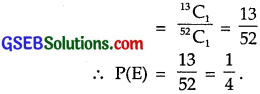There are four aces in a deck of cards.
∴ Probability of drawing an ace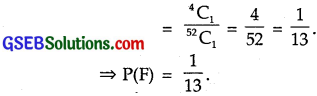There is only one card which is an ace of spade
∴ Probability of drawing an ace of spade = $$\frac{1}{52}$$.
∴ P(E ∩ F) = $$\frac{1}{52}$$ = $$\frac{1}{4}$$ × $$\frac{1}{13}$$ = P(E) × P(F)
⇒ P(E ∩ F) = P(E) × P(F).
⇒ Events E and F are independent.

(ii) There are 26 black cards in a deck of 52 cards.
∴ Probability of drawing 1 black card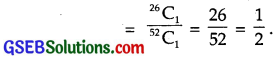∴ P(E) = $$\frac{1}{2}$$
There are 4 king cards out of 52 cards of the deck
∴ Probability of drawing a king.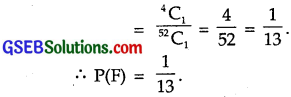These are tow kings of black colour.
∴ Probability of drawing a king of black colour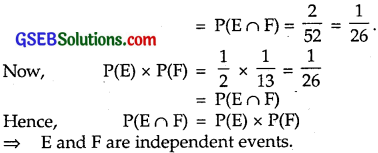(iii) These are 4 king and 4 queen cards.
∴ Probability of drawing a king or queen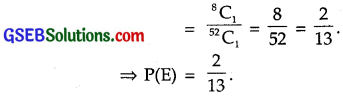There are 4 queen and 4 jack cards.
∴ Probability of drawing a queen or a jack = $$\frac{8}{52}$$ = $$\frac{2}{13}$$.
Out of 4 kings and 4 queens; 4 queens and 4 jacks,
there are 4 queens common in both cases.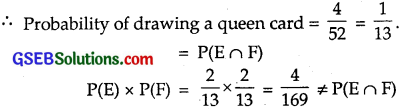Here, E and F are not independent events.Question 16.
In a hostel, 60% of the students read Hindi newspaper, 40% read English newspaper and 20% read both Hindi and English newspapers.
A student is selected at random.
(a) Find the probability that she read neither Hindi nor English newspaper.
(b) If she reads Hindi newspaper, find the probability that she reads English newspaper also.
(c) If she reads English newspaper, find the probability that she reads Hindi newspaper also.
Solution:
(a) Let H and E represent the event that a student reads Hindi and English newspapers respectively.
∴ P(H) = 0.6, P(E) = 0.4, P(H ∩ E) = 0.2
Probability that the students reads at least one paper
= P(H ∪ E)
Now, P(H) = 0.6 + 0.4 – 0.2 = 1 – 0.2 = 0.8.
∴ Probability that a student reads neither Hindi nor English newspaper
= 1 – P(H ∪ E) = 1 – 0.8 = 0.2

(b) The probability that the student reads English newspaper if she reads Hindi newspaper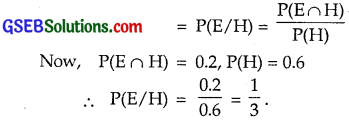(c) The probability that she reads Hindi newspaper, if she reads English newspaper = P(H/E)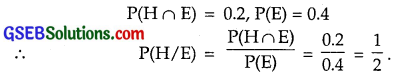Choose the correct answers in questions 17 and 18:

Question 17.
The probability of obtaining an even prime number on each die, when a pair of dice is rolled is
(A) 0
(B) $$\frac{1}{3}$$
(C) $$\frac{1}{12}$$
(D) $$\frac{1}{36}$$
Solution:
There are only one even prime number, i.e., 2, when a dice is rolled, the probability of getting an even prime number = $$\frac{1}{6}$$.
When two dice are rolled, the probability of getting an even prime number on each dice = $$\frac{1}{6}$$ × $$\frac{1}{6}$$ = $$\frac{1}{36}$$.
∴ Part (D) is the correct answer.Question 18.
Two events A and B are said to be independent, if
(A) A and B are mutually exclusive.
(B) P(A’B’) = [1 – P(A)] [1 – P(B)]
(C) P(A) = P(B)
(D) P(A) + P(B) = 1
Solution:
Two events are independent,
if P(A ∩ B) = P(A) × P(B)
or P(A’ ∩ B’) = P(A’) P(B’) = [1 – P(A)][1 – P(B)]
⇒ Part (B) is the correct answer.